# Place Value with Base Ten Blocks (MAB / Dienes Blocks)

Place Value with Base Ten Blocks (MAB / Dienes Blocks)• Base 10 blocks are also called Dienes blocks, Multibase Arithmetic blocks (MAB blocks) or Place Value blocks.
• The four types of base ten blocks are named units, rods, flats and cubes.
• The unit cubes are worth 1, the long rods are worth 10, the flats are worth 100 and the cube blocks are worth 1000.
• The blocks are called base ten because ten of each block can be replaced with another, larger block.
• Base 10 blocks are used for teaching place value, regrouping and they can be helpful for teaching volume.
• Base 10 blocks are often used to teach place value by placing them inside a blank place value chart.

Base Ten Blocks are used to teach place value and represent numbers physically.

Units are worth 1, Rods are worth 10, Flats are worth 100 and Cubes are worth 1000.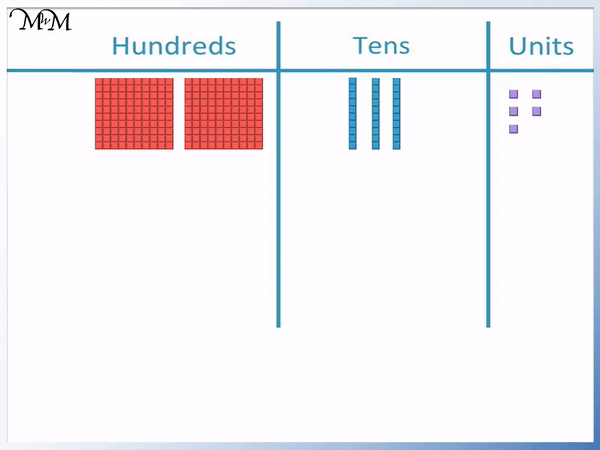• The base ten blocks are used to represent numbers by grouping them into place value columns on a blank place value chart.
• There are 2 flat blocks in the hundreds column, so we can write a ‘2’ digit.
• There are 3 rod blocks on the tens column, so write a ‘3’ digit.
• There are 5 unit blocks in the units/ones column, so write a ‘5’ digit.
• These base ten blocks are used to model the number 235.# Using Base Ten Blocks (MAB / Dienes) Blocks to Teach Place Value

## What are Base 10 Blocks?

Base ten blocks are also known as Dienes blocks, Multibase Arithmetic Blocks (MAB Blocks for short) and Place Value blocks.

Base 10 blocks are objects that are used to represent Units (Ones), Tens, Hundreds and Thousands. They are made up of small cubes.

There are four types of base ten blocks, which are called the unit block, the rod, the flat and the cube block.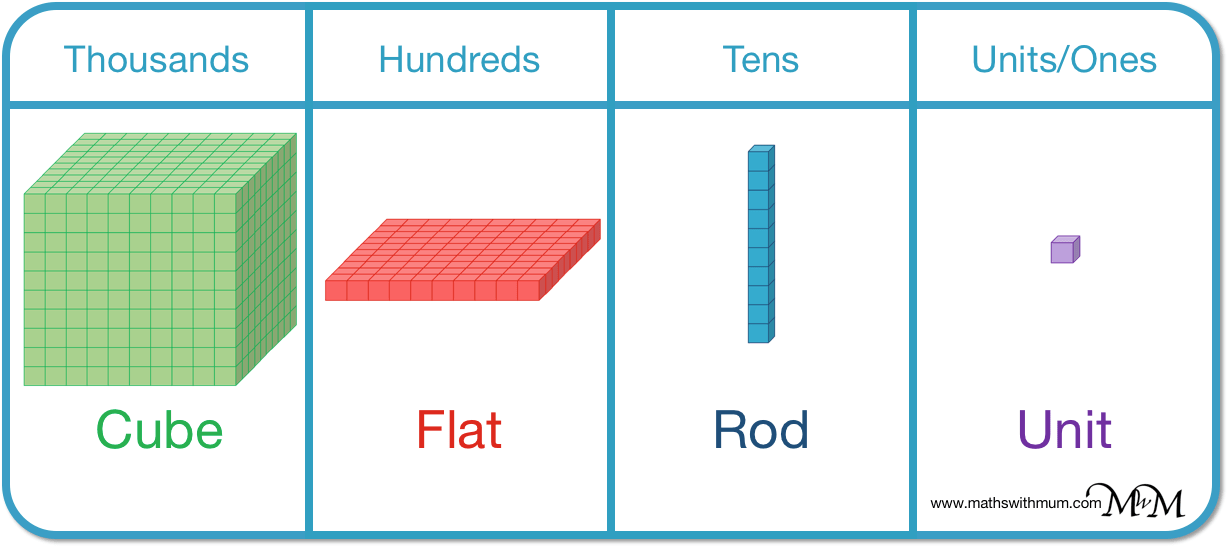The smallest value is one cube unit block, which is worth 1.A row of ten unit cubes are joined together to make a rod.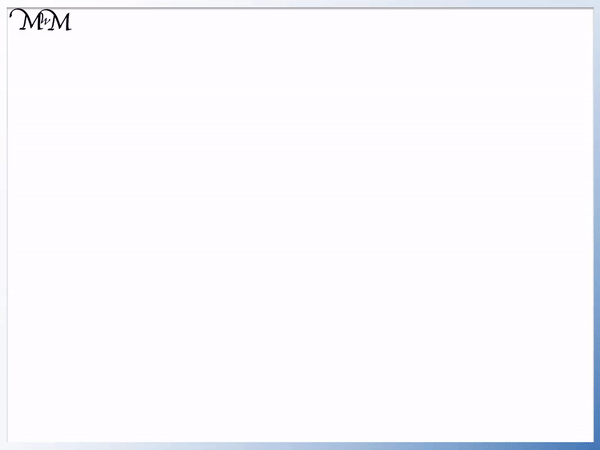The rod (or long) is worth 10.Ten rows of these rods can be joined together to make a flat sheet of 100 unit cubes.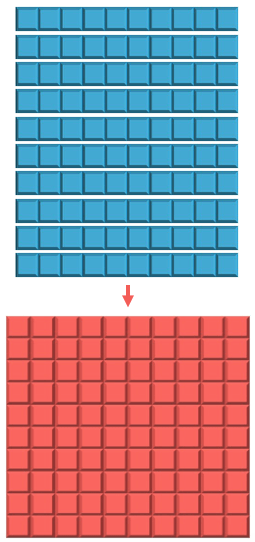The flat is worth 100.Ten flats can be joined together to make a cube block.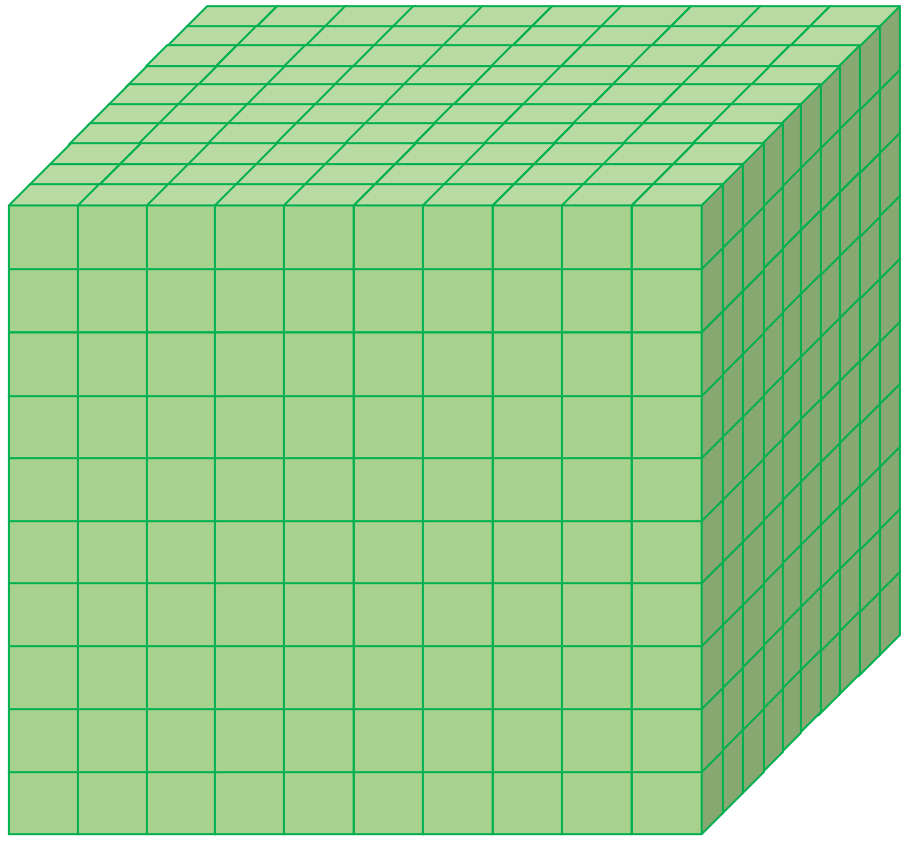The cube block is worth 1000. This is the MAB base ten block with the largest value.

Below is a printable teaching poster that may be useful for teaching the Dienes block values.

Dienes blocks (or MAB blocks) are also called base 10 blocks because they follow our number and place value system. This system is called the base ten system because the numbers are built from 10 different digits: 1, 2, 3, 4, 5, 6, 7, 8, 9 and 0. This means that each place value column works in groups of ten. The value of each place value column is ten times greater than the one to its right. We could also say that the value of each place value column is ten times smaller than the one to its left.## Why are Base 10 Blocks Important?

Base 10 blocks are a useful way to enable children to visualise numbers and to see how they relate to each other in our number system. Base ten Dienes blocks are a physical manipulative that children can used to build and represent numbers. The advantage of Dienes blocks is that they can easily represent larger numbers when compared to other manipulatives such as counters.

MAB base ten blocks allow children to see how ten unit blocks in the units column can be replaced with one ten rod, which we can then move into the tens column. This helps children to build a stronger understanding of the regrouping process which is used in addition and subtraction.

## How to use Base 10 Blocks to Represent, Model and Build Numbers

Base ten MAB blocks can be used to represent numbers by placing them in groups onto a blank place value chart. The number of each types of base ten block is the same as the digits of the number being represented.

When teaching base ten place value, it is best to start with the units column and work through the columns looking at larger and larger numbers.

Here are some unit cube MAB blocks used to represent the first few counting numbers.Here are 3 unit blocks.Units are the smallest value of the Dienes blocks and we put these three blocks in the units column of the place value chart.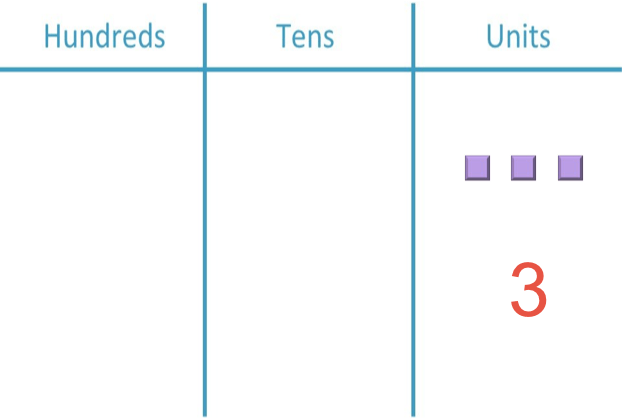The 3 units are simply worth 3.

Here is an example of drawing the number 6 with base ten blocks.Which number is represented by the base ten block image example below?We put the Dienes blocks onto a blank place value chart. The rods are worth ten and so they belong in the tens column.The number drawn in the image above contains 4 rods in the tens column. We write a ‘4’ in the tens column.

There are no blocks in the units column and so we put a ‘0’.

We have 40 represented with the base ten blocks.

Here are some examples of numbers drawn in base ten blocks for you to work out the value of.We have 3 ten rods drawn as base ten blocks.

3 tens are worth 30.

These MAB base ten blocks are worth 30.

Here are some Dienes rod blocks drawn on a place value chart. What is the value of these blocks?We have 5 ten rods. 5 tens are 50.

We will now try drawing numbers with base ten blocks.

Try drawing 500 using base ten blocks.

Looking at the digits from right to left, we have ‘0’, ‘0’ and ‘5’.

We need ‘0’ units, ‘0’ tens but ‘5’ hundreds. We pick up 5 of the hundred flats.5 hundred flat Dienes blocks are worth 500.

What is the value of the MAB base ten blocks shown in the following place value chart?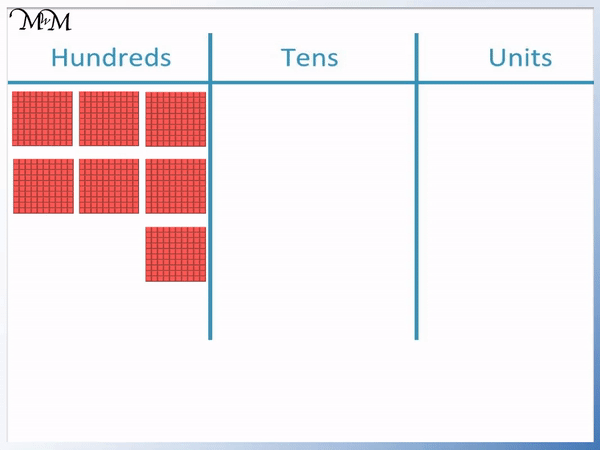We have 7 hundred flat MAB blocks.

7 hundreds are worth 700.

We can write this number with a 7 in the hundreds column because there are 7 separate Dienes blocks in the hundreds column.

We write a zero in the other columns because there is nothing in them.

We will now look at some examples of drawing and modelling numbers that are built from more than one type of base ten block.

What is the 3-digit number represented by this set of base ten blocks?We count the number of MAB blocks in each place value column.

In the hundreds column we have 2 blocks.

In the tens column we have 3 blocks.

In the units / ones column we have 5 blocks.

The number represented by these base ten blocks is 235.Here is another example of modelling a number using Dienes bas ten blocks.There are 4 hundred flats, 2 ten rods and 8 unit cubes.

The number that has been built is 428.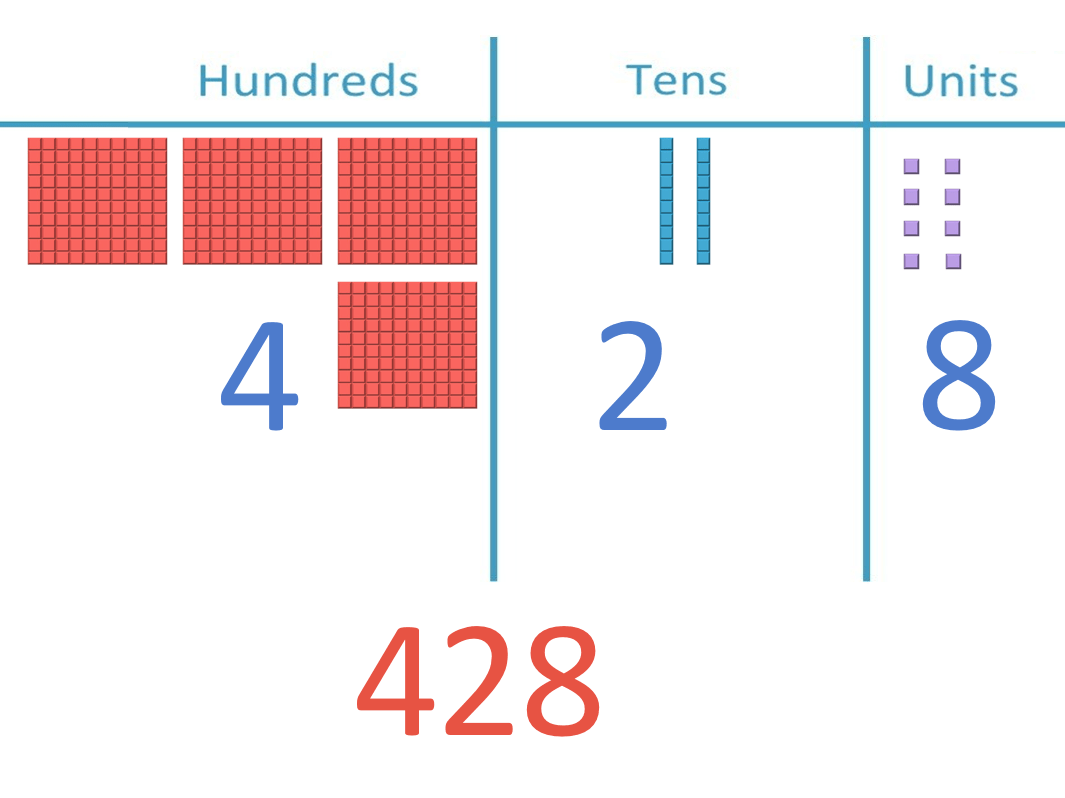We will now try drawing the number 890 using place value blocks.

Looking at the digits from right to left, 890 contains ‘0’ unit blocks, ‘9’ tens rods and ‘8’ hundred flats.What number is drawn using the Dienes base ten blocks shown?There are ‘7’ unit blocks, ‘0’ tens rods and ‘6’ hundred flats.

We have 607.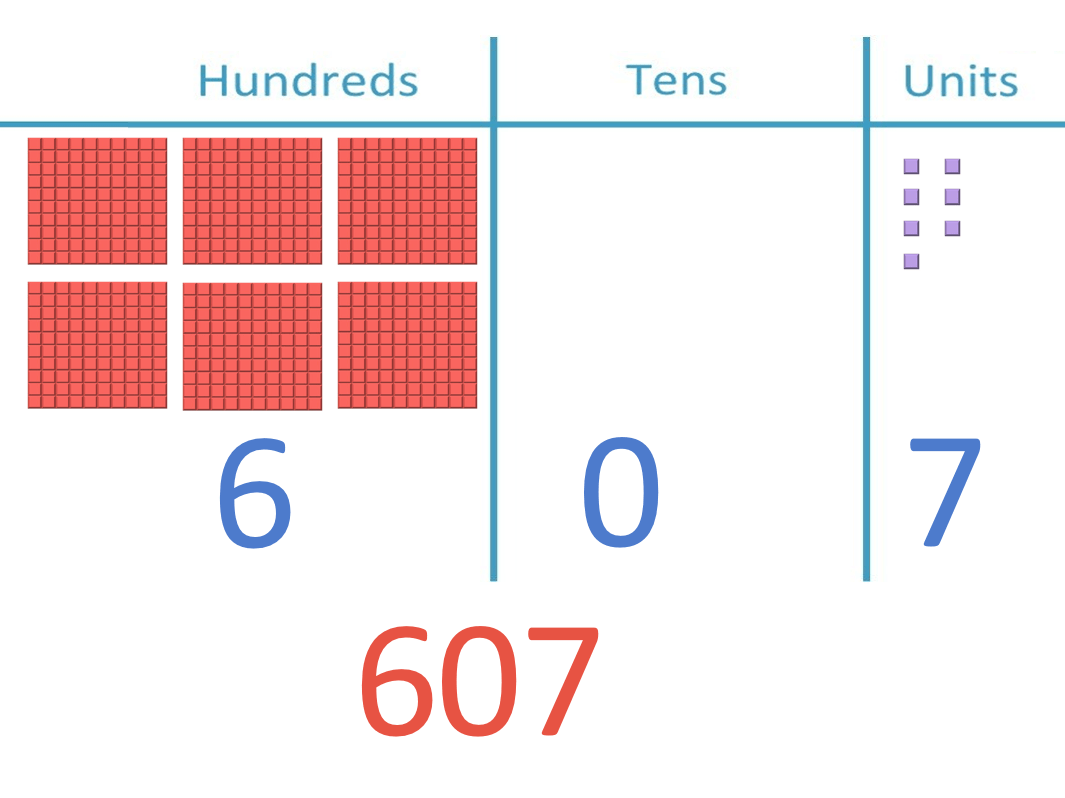We have a printable place value chart for teaching place value using base ten blocks to work along with all of these examples.

## What are some other uses for base 10 blocks?

Base 10 blocks are useful when introducing addition and subtraction using written methods such as column addition. They can help to visualise the size of the numbers and are a useful teaching tool for introducing these topics.

Our lessons using place value blocks for teaching addition and subtraction can be found at:

Place value blocks are also useful for understanding the regrouping process used in addition and subtraction. Regrouping with base 10 blocks has been seen already where we exchange 10 units for a tens rod or 10 tens rods for a one hundred flat piece.

Base 10 blocks can also be used to introduce volume. The unit cubes can be used to show the unit value (simplest value), which would be one centimetre cubed (cm3). These can then be used to show how they can be combined to make different sizes of cubes, cuboids or other abstract shapes. The volume of the new shape can be found by counting up the total number of cubes.Now try our lesson on Regrouping with Base Ten Blocks where we learn how to regroup using base ten blocks.error: Content is protected !!